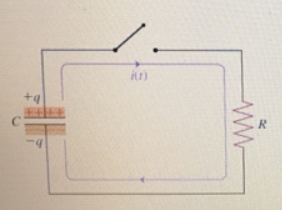# Problem: Learning Goal: To study the behavior of a circuit containing a resistor and a charged capacitor when the capacitor begins to discharge.  A capacitor with capacitance C is initially charged with charge q. At time t = 0, a switch is thrown to close the circuit connecting the capacitor in series with a resistor of resistance R (Figure 1) What is the current I0 that follows through the resistor immediately after the switch is thrown?

###### FREE Expert Solution

Ohm's law:

$\overline{){\mathbf{V}}{\mathbf{=}}{\mathbf{i}}{\mathbf{R}}}$

The charge stored in a capacitor:

$\overline{){\mathbf{Q}}{\mathbf{=}}{\mathbf{C}}{\mathbf{V}}}$

100% (147 ratings)###### Problem Details

Learning Goal: To study the behavior of a circuit containing a resistor and a charged capacitor when the capacitor begins to discharge.

A capacitor with capacitance C is initially charged with charge q. At time t = 0, a switch is thrown to close the circuit connecting the capacitor in series with a resistor of resistance R (Figure 1)What is the current I0 that follows through the resistor immediately after the switch is thrown?